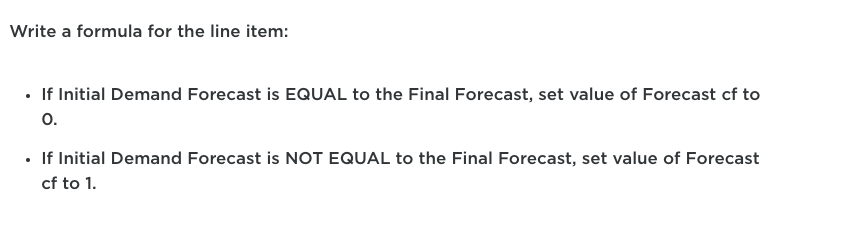# Formula for Forecast cfI was working on the formula for Forecast cf and wrote out this formula as instructed."IF Initial Demand Forecast = Final Forecast THEN Forecast cf = 0 ELSE Forecast cf = 1"

However, I am met with an error stating that Forecast cf line item has a number format, but the formula is question resolves to a Boolean format. Changing the forecast cf line item format to Boolean is not the answer so I was wondering what was wrong with my formula?